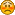###Author Topic: robot arm desaigner  (Read 2167 times)

0 Members and 1 Guest are viewing this topic.

####wicaksono• Beginner
•• Posts: 2##### robot arm desaigner
« on: September 05, 2013, 05:51:27 PM »
Hi there ! Hi Admin ! Hi guest !I have a question for robot arm desaigner (excel sheet:  Robot Arm Designer v1) especially about forward kinematics.

On  Robot Arm Designer v1, there have 2 joints with equations :

Joint 0 (with x and y at base equaling 0):
x0 = 0
y0 = L0

Joint 1 (with x and y at J1 equaling 0):
cos(psi) = x1/L1 => x1 = L1*cos(psi)
sin(psi) = y1/L1 => y1 = L1*sin(psi)

Joint 2 (with x and y at J2 equaling 0):
sin(theta) = x2/L2 => x2 = L2*cos(theta)+x1
cos(theta) = y2/L2 => y2 = L2*sin(theta)+y1

What if I made equation for 3 joints?
And then I made equation for 3 joints like this :

Joint 3
x3 = L3*cos(theta)+x1+x2
y3 = L3*sin(theta)+y1+y2

Was it right ?
Please help me.oh one more.On excel sheet, value of theta (x2 and y2) is theta1 + theta2. Why ?

####nrox

• Jr. Member
•• Posts: 8##### Re: robot arm desaigner
« Reply #1 on: September 05, 2013, 07:00:15 PM »
Do you consider using AI to control robot arms? I am doing some experiments with that:
https://assemblino.com/show/public20123372.html
Requires Chrome and WebGL to see the live project.

####wicaksono• Beginner
•• Posts: 2##### Re: robot arm desaigner
« Reply #2 on: September 07, 2013, 01:14:43 AM »
@nrox : next time.

How about my question, can you solve it?

####nrox

• Jr. Member
•• Posts: 8##### Re: robot arm desaigner
« Reply #3 on: September 12, 2013, 09:40:03 AM »
Hi,
I studied mech eng some years ago, and I know that robot arm programming is a pita. That's why I am interested in alternative methods.

Your question would be probably solved with this:

Joint 3
x3 = L3*cos(theta)+x2
y3 = L3*sin(theta)+y2

if it's the last joint. because you take the reference from the one before.

Can you give me some feedback about assemblino.com and the method for trainning arms with NN ? I would appreciate that. The software is new and I need feedback to improve it, or just trow it into the trash bin.